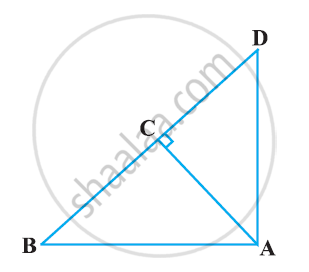# In Figure, ABD is a triangle right angled at A and AC ⊥ BD. Show that AB^2 = BC × BD - Mathematics

In Figure, ABD is a triangle right angled at A and AC ⊥ BD. Show that AB2 = BC × BD#### Solution

In ΔADB and ΔCAB, we have

∠DAB = ∠ACB (Each equals to 90°)

∠ABD = ∠CBA (Common angle)

∴ ΔADB ~ ΔCAB [AA similarity criterion]

⇒(AB)/(CB) = (BD)/(AB)

⇒ AB2 = CB × BD

Concept: Right-angled Triangles and Pythagoras Property
Is there an error in this question or solution?

#### APPEARS IN

NCERT Class 10 Maths
Chapter 6 Triangles
Exercise 6.5 | Q 3.1 | Page 150